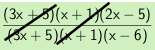### Home > AC > Chapter 11 > Lesson 11.1.4 > Problem11-50

11-50.

Simplify each expression below.

1. $\frac{3x^2+8x+5}{x^2-5x-6}\cdot\frac{2x-5}{3x+5}$

Factor, then multiply.

$\frac{\left(3x+5\right)\left(x+1\right)\left(2x-5\right)}{\left(3x+5\right)\left(x+1\right)\left(x-6\right)}$

Look for factors that make $1$.$\frac{\left(2x-5\right)}{\left(x-6\right)}$

1. $\frac{x^2+x-12}{x^2-x-6}\div\frac{x-5}{x^2-3x-10}$

Change the division to multiplication, then see part (a).